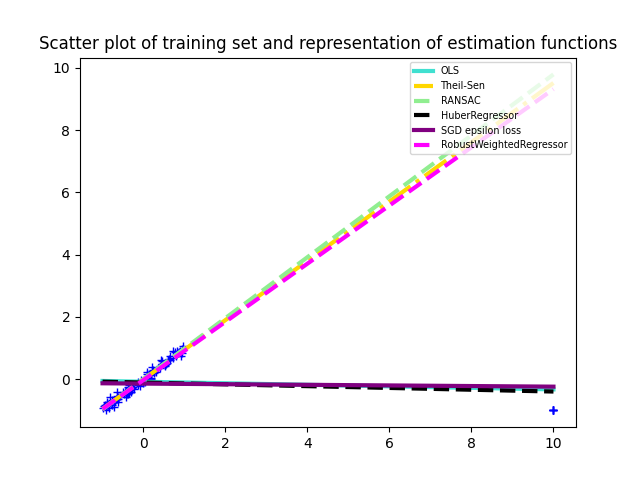# Robust regression on simulated corrupted dataset¶

In this example we compare the RobustWeightedRegressor with various robust regression algorithms from scikit-learn.```import matplotlib.pyplot as plt
import numpy as np

from sklearn_extra.robust import RobustWeightedRegressor
from sklearn.utils import shuffle
from sklearn.linear_model import (
SGDRegressor,
LinearRegression,
TheilSenRegressor,
RANSACRegressor,
HuberRegressor,
)

# Sample along a line with a Gaussian noise.
rng = np.random.RandomState(42)
X = rng.uniform(-1, 1, size=)
y = X + 0.1 * rng.normal(size=100)

# Change the 5 last entries to an outlier.
X[-5:] = 10
X = X.reshape(-1, 1)
y[-5:] = -1

# Shuffle the data so that we don't know where the outlier is.
X, y = shuffle(X, y, random_state=rng)
estimators = [
("OLS", LinearRegression()),
("Theil-Sen", TheilSenRegressor(random_state=rng)),
("RANSAC", RANSACRegressor(random_state=rng)),
("HuberRegressor", HuberRegressor()),
(
"SGD epsilon loss",
SGDRegressor(loss="epsilon_insensitive", random_state=rng),
),
(
"RobustWeightedRegressor",
RobustWeightedRegressor(weighting="mom", k=7, random_state=rng),
# The parameter k is set larger to the number of outliers
# because here we know it.
),
]

colors = {
"OLS": "turquoise",
"Theil-Sen": "gold",
"RANSAC": "lightgreen",
"HuberRegressor": "black",
"RobustWeightedRegressor": "magenta",
"SGD epsilon loss": "purple",
}
linestyle = {
"OLS": "-",
"SGD epsilon loss": "-",
"Theil-Sen": "-.",
"RANSAC": "--",
"HuberRegressor": "--",
"RobustWeightedRegressor": "--",
}
lw = 3

x_plot = np.linspace(X.min(), X.max())

plt.plot(X, y, "b+")

for name, estimator in estimators:
estimator.fit(X, y)
y_plot = estimator.predict(x_plot[:, np.newaxis])
plt.plot(
x_plot,
y_plot,
color=colors[name],
linestyle=linestyle[name],
linewidth=lw,
label="%s" % (name),
)

legend = plt.legend(loc="upper right", prop=dict(size="x-small"))

plt.title(
"Scatter plot of training set and representation of"
" estimation functions"
)
plt.show()
```

Total running time of the script: ( 0 minutes 0.148 seconds)

Gallery generated by Sphinx-Gallery